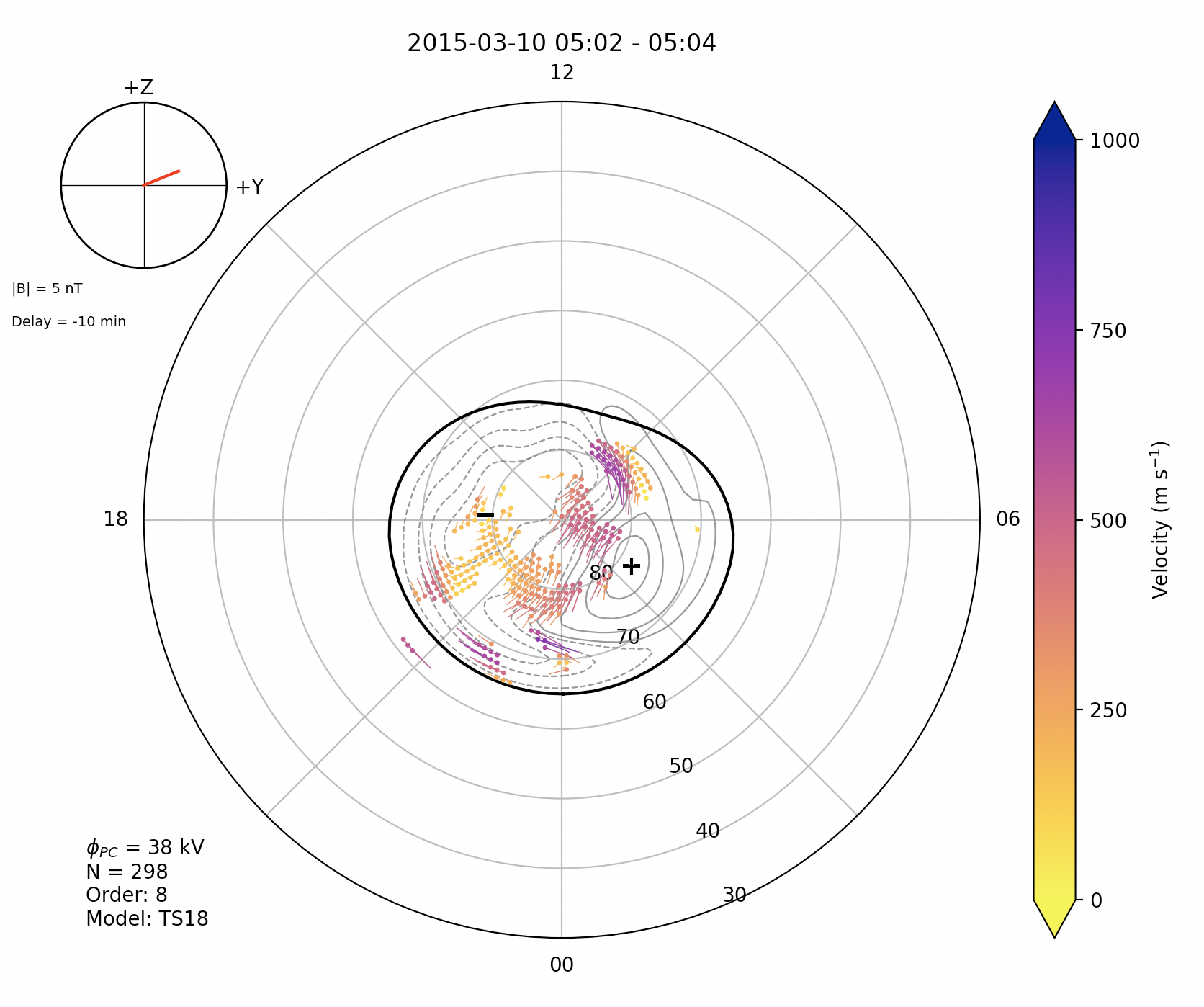# Map plots

Map plots are a way to visualize data from a MAP file of SuperDARN radar data. Please read RST documentation on how to process MAP files from GRID files.

Map field descriptions can be found here. pyDARN uses a `enum` object to select different common parameters to plot in a MAP file:

Name parameter name Map field name
Fitted Velocity `MapParams.FITTED_VELOCITIES` see Fitted Velocities section
Modeled Velocities `MapParams.MODEL_VELOCITIES` `model.vel.median`
Raw Velocities `MapParams.RAW_VELOCITIES` `vector.vel.median`
Power `MapParams.POWER` `vector.pwr.median`
Spectral Width `MapParams.SPECTRAL_WIDTH` `vector.wdt.median`

for a given `start_time` or `record` number projected onto a polar plot in AACGMv2 coordinates.

Currently, map plots in pyDARN get geomagnetic positions of the mapped data in `mlon` and `mlat` from the MAP file, which uses AACGMv2 coordinates. In the future, pyDARN will also generate the geographic position of the data points, which will bring support for non-standard gridded vector layouts.

### Fitted Velocities

Fitted velocities are velocity vectors which represent the fitted convection pattern. They are entirely in the direction of the fitted convection flow and so can be fairly different to line-of-sight velocities, but still ultimately constrained by them.

Fitted velocity vectors are by default only calculated at the same positions of the line-of-sight vectors, but fit vectors at an arbitrary position can be obtained by using the `calculated_fitted_velocities` function in `map.py`.

## Basic usage

pyDARN and pyplot need to be imported and the desired MAP file needs to be read in:

``````import matplotlib.pyplot as plt
import pydarn

file = "path/to/grid/file"

``````

With the map data loaded as a list of dictionaries (`map_data` variable in above example), you may now call the `plot_mapdata` method. Make sure you tell the method what time, in [`datetime` format], or record number (numbered from first recorded in file, counting from 0):

``````mapplot = pydarn.Map.plot_mapdata(map_data, record=150)
plt.show()

``````

In this example, the record at 150 was plotted with the defaulted parameter, `MapParams.FITTED_VELOCITIES` (fitted velocities), being mapped:You might have noticed that the variable `mapplot` in the examples above actually holds some information. This contains the AACGM latitude and longitude of the mapped vectors plotted. If you instead change `mapplot` to 3 separate variables, it will return the latitude, longitude, and data info into separate variables:

``````lats,lons,data=pydarn.Map.plot_mapdata(map_data, start_time=stime)
``````

Here is a list of all the current options than can be used with `plot_mapdata`

Option Action
record=(int) Record number to plot
start_time=(datetime.datetime) The start time of the record to plot
time_delta=(int) How close to the start time to be to the start time of the record
parameter=(Enum) Specify the required parameter described above i.e. `pydarn.MapParams.FITTED_VELOCITY`
lowlat=(int) Control the lower latitude boundary of the plot (default 30/-30 AACGM lat)
len_factor=(int) Normalisation factor for the length of the vectors on the plot
boundary=(bool) Boolean to show the Field-of-View of the radar(s)
cmap=matplotlib.cm A matplotlib color map object. Will override the pyDARN defaults for chosen parameter
zmin=(int) Minimum data value for colouring
zmax=(int) Maximum data value for colouring
colorbar=(bool) Set true to plot a colorbar (default: True)
colorbar_label=(string) Label for the colour bar (requires colorbar to be true)
title=(str) To add a title to the plot
hmb=(bool) Set to True to include the Heppnar-Maynard Boundary on the plot
imf_dial=(bool) Show the IMF data as a clock angle dial (default True)
map_info=(bool) Show selected map file data (e.g. cross polar cap potential) (default True)
contour_levels=(list: floats) Set custom levels for contours to appear at
contour_color=(str) Set custom color for contour lines (default 'dim grey')
contour_linewidths=(float) Set custom line width for contour lines (default 0.8)
contour_fill=(bool) Choose to use filled contours, default is False to plot contour lines only
contour_colorbar=(bool) If contour_fill=True, color bar will be displayed (default True)
contour_fill_cmap=(str) If contour_fill=True, color map can be selected (default 'RdBu')
contour_colorbar_label=(str) If contour_fill and contour_colorbar= True, set custom contour colorbar label
pot_minmax_color=(str) Choose color of minimum and maximum potential markers

More `**kwargs` can be used to customise the display of the radars field-of-view if `boundary=True`

The following is an example of the customization available:

``````import matplotlib.pyplot as plt
import pydarn

map_file = "20150310.n.map"

pydarn.Maps.plot_mapdata(map_data, record=150,
parameter=pydarn.MapParams.FITTED_VELOCITY,
lowlat=60, colorbar_label='Velocity m/s',
contour_fill = True,
contour_fill_cmap= 'RdBu',
contour_colorbar = True,
contour_colorbar_label='Potential (kV)',
pot_minmax_color = 'r',
map_info=True, imf_dial=True, hmb=True)

plt.show()
``````